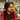# LFU Cache

This is a follow up question to LRU cache which is covered Here

What’s an LFU Cache:

• A system to maintain a cache with in a limited amount of memory.
• If the memory is limited, obviously we’ll reach a point where there is no space left to store the cache item.
• So the least recently used (LFU) item is removed from the cache when the cache is full.
• We maintain a map to store the frequency of each key used in the cache and remove the key with the least frequency when the memory fills.
• We also maintain the position of the cache item which was used the earliest, just like we do in LRU Cache.
• In case there is a clash between the keys to be removed which will happen if multiple keys have the same least frequency, the least recently used key among the candidates is removed.
• So in a way, LFU required LRU cache implementation also.
• Valid operations on a cache system:

1. get(key)
2. put(key, value)

## Example:

``````Cache capacity: 2
put(1,1)    //Cache: {1:1},         Frequency: {1:1}
put(2,2)    //Cache: {1:1, 2:2},    Frequency: {1:1, 2:1}
get(1)      //Cache: {1:1, 2:2},    Frequency: {1:2, 2:1},      Output: 1
put(3,3)    //Cache: {1:1, 3:3},    Frequency: {1:2, 3:1}
get(2)      //Cache: {1:1, 3:3},    Frequency: {1:2, 3:1},      Output: -1
get(3)      //Cache: {1:1, 3:3},    Frequency: {1:2, 3:2},      Output: 3
put(4,4)    //Cache: {3:3, 4:4},    Frequency: {3:2, 4:1} <-- Remove 1 because it was least recently used among 1 & 3
get(1)      //Cache: {3:3, 4:4}     Frequency: {3:3, 4:4},      Output: -1
get(3)      //Cache: {3:3, 4:4},    Frequency: {3:3, 4:1},      Output: 3
get(4)      //Cache: {3:3, 4:4},    Frequency: {3:3, 4:2},      Output: 4``````

## Implementation

Here we will maintain a map frequency to maintain the frequency of each key used.
Also we’ll maintain an array history in which the items will be sorted according to their most recent occurrence.

``````var LFUCache = function (capacity) {
this.size = capacity
this.frequency = new Map()
this.map = new Map()
this.history = []
}

LFUCache.prototype.get = function (key) {
if (!this.map.has(key)) return -1

this.frequency.set(key, this.frequency.get(key) + 1)
this.updateHistory(key)
return this.map.get(key)
}

LFUCache.prototype.put = function (key, value) {
if (this.size == 0) return
if (this.map.has(key)) {
this.frequency.set(key, this.frequency.get(key) + 1)
this.updateHistory(key)
this.map.set(key, value)
} else {
if (this.map.size >= this.size) {
var leastFrequency = Math.min(...this.frequency.values())

var candidates = []
for (var frequencyKey of this.frequency.keys()) {
if (this.frequency.get(frequencyKey) == leastFrequency) {
candidates.push(frequencyKey)
}
}

var keyToDelete = candidates
if (candidates.length > 1) {
var leastIndex = Infinity
for (var candidate of candidates) {
var tempIndex = this.history.indexOf(candidate)
if (tempIndex != -1 && tempIndex < leastIndex) {
keyToDelete = candidate
leastIndex = tempIndex
}
}
this.history.splice(leastIndex, 1)
}

this.map.delete(keyToDelete)
this.frequency.delete(keyToDelete)
}

this.map.set(key, value)
this.frequency.set(key, 1)
this.updateHistory(key)
}
}
LFUCache.prototype.updateHistory = function (key) {
var index = this.history.indexOf(key)
if (index != -1) this.history.splice(index, 1)
this.history.push(key)
}``````

## Execution:

``````var lfu = new LRUCache(2)

lfu.put(1, 1)
lfu.put(2, 2)
lfu.get(1)
lfu.put(3, 3)
lfu.get(2)
lfu.get(3)
lfu.put(4, 4)
lfu.get(1)
lfu.get(3)
lfu.get(4)``````Written by Gagandeep Rangi who likes to talk about himself in third person. Twitter Instagram